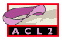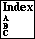## DEFTHM

prove and name a theorem
```Major Section:  EVENTS
```

```Examples:
(defthm assoc-of-app
(equal (app (app a b) c)
(app a (app b c))))
```
The following nonsensical example illustrates all the optional arguments but is illegal because not all combinations are permitted. See hints for a complete list of hints.
```(defthm main
(implies (hyps x y z) (concl x y z))
:rule-classes (:REWRITE :GENERALIZE)
:instructions (induct prove promote (dive 1) x
(dive 2) = top (drop 2) prove)
:hints (("Goal"
:do-not '(generalize fertilize)
:in-theory (set-difference-theories
(current-theory :here)
'(assoc))
:induct (and (nth n a) (nth n b))
:use ((:instance assoc-of-append
(x a) (y b) (z c))
(:functional-instance
(:instance p-f (x a) (y b))
(p consp)
(f assoc)))))
:otf-flg t
:doc "#0[one-liner/example/details]")

General Form:
(defthm name term
:rule-classes rule-classes
:instructions instructions
:hints        hints
:otf-flg      otf-flg
:doc          doc-string)
```
where `name` is a new symbolic name (see name), `term` is a term alleged to be a theorem, and `rule-classes`, `instructions`, `hints`, `otf-flg` and `doc-string` are as described in their respective documentation. The five keyword arguments above are all optional, however you may not supply both `:``instructions` and `:``hints`, since one drives the proof checker and the other drives the theorem prover. If `:``rule-classes` is not specified, the list `(:rewrite)` is used; if you wish the theorem to generate no rules, specify `:``rule-classes` `nil`.

When ACL2 processes a `defthm` event, it first tries to prove the term using the indicated hints (see hints) or instructions (see proof-checker). If it is successful, it stores the rules described by the rule-classes (see rule-classes), proving the necessary corollaries.